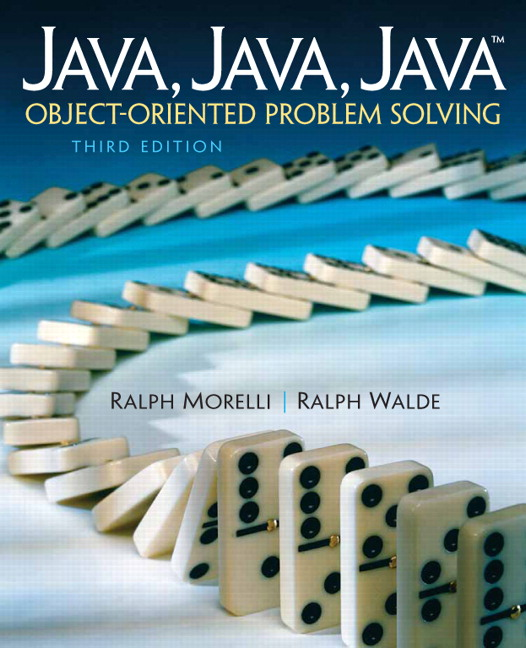# Java, Java, Java: Object-Oriented Problem Solving, 2022E

## Section12.6OBJECT-ORIENTED DESIGN: Tail Recursion

As we saw in the previous section, it is relatively simple to convert the drawBoxes() method into an iterative version:
private void drawBoxesIterative(Graphics g, int level, int locX, int locY, int side, int delta) {
for (int k = level; k >= 0; k--) {
g.drawRect(locX, locY, side, side); // Draw a square
locX += delta; // Calculate new location
locY += delta;
side -= 2 * delta; // Calculate new side length
}
} // drawBoxesIterative()

private void drawBoxes(Graphics g, int level, int locX, int locY, int side, int delta) {
g.drawRect(locX, locY, side, side);
if (level > 0) {
int newLocX = locX + delta;
int newLocY = locY + delta;
drawBoxes(g, level - 1, newLocX, newLocY, side - 2 * delta, delta);
} // if
} // drawBoxes()

However, the same cannot be said for the drawGasket() method. It is clearly a case where the recursive approach makes the problem easier to solve.
One difference between drawBoxes() and drawGasket() is that drawBoxes() is an example of a tail-recursive method. A method is tail recursive if all of its recursive calls occur as the last action performed in the method. You have to be a bit careful about this definition. The recursive call in a tail-recursive method has to be the last executed statement. It needn’t be the last statement appearing in the method’s definition.
For example, the following method will print “Hello” N times. This method is tail recursive even though its last statement is not a recursive call:
public void printHello(int N) {
if (N > 1) {
System.out.println("Hello");
printHello(N - 1); // The last executed statement
} else
System.out.println("Hello");
} // printHello()

This method is tail recursive because the last statement that will be executed, in its recursive cases, is the recursive call.
A tail-recursive method is relatively easy to convert into an iterative method. The basic idea is to make the recursion parameter into a loop variable, taking care to make sure the bounds are equivalent. Thus, the following iterative method will print “Hello” N times:
public void printHelloIterative(int N) {
for (int k = N; k > 0; k--)
System.out.println("Hello");
}

In this case, we use the parameter N to set the initial value of the loop variable, k, and we decrement k on each iteration. This is equivalent to what happens when we decrement the recursion parameter in the recursive call.
As you can see, recursive methods that are not tail recursive are much more complex. Just compare the drawGasket() and drawBoxes() methods. Yet it is precisely for these nontail-recursive algorithms that recursion turns out to be most useful.
As you might expect, if you can’t give a simple tail-recursive solution to a problem, the problem probably doesn’t have a simple iterative solution either. Thus, the problems where we most need recursion are those where we can’t give a simple tail-recursive or a simple iterative solution. And there are a lot of such problems, especially when you get into nonlinear data structures such as trees and graphs.
To gain some appreciation for this complexity, consider how difficult it would be to draw the Sierpinski gasket using an iterative approach. We could start by developing an outer for loop to account for the different levels in the pattern:
for (int k = lev; k > 0; k--) {
drawGasket(g, lev - 1, p1X, p1Y, q1X, q1Y, q2X, q2Y);
drawGasket(g, lev - 1, p2X, p2Y, q1X, q1Y, q3X, q3Y);
drawGasket(g, lev - 1, p3X, p3Y, q2X, q2Y, q3X, q3Y);
}

But now each of the method calls within the body of this loop would have to be replaced by very complex loops. That would be a daunting task. So the lesson to be drawn from this observation is that recursion is most useful as a problem-solving technique for problems that don’t yield to a simple iterative solution.

### ExercisesSelf-Study Exercises

#### 2.countChar() tail recursive?

True or false, the countChar() method is tail recursive.
public int countChar(String s, char ch) {
if (s.length() == 0)    // Base case: empty string
return 0;
else if (s.charAt(0) == ch)  // Recursive case 1
return 1 + countChar(s.substring(1), ch); // Head = ch
else                         // Recursive case 2
return 0 + countChar(s.substring(1), ch); // Head != ch
} // countChar()

• True
• Right, the recursive call IS the last statement to be executed.
• False
• Is the recursive call the last statement to be executed?